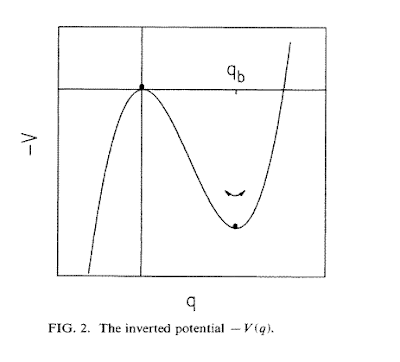## Wednesday, July 15, 2009

### Over the top or tunnel through? Quantum reaction rate theory

A major theoretical challenge is to calculate the rate of a chemical reaction.
Most reactions proceed by a rearrangement of the geometrical arrangement of the atoms in the reactant molecules leading to the product molecules. This can be described by a potential energy surface (PES) in which the reactants and pproduct configurations are local minima (R and P, respectively) separated by some saddle point known as the transition state (TS). The concept of the TS was introduced by Eyring in 1935. In the Figure below R is at q=0.The difference in energy between the TS and the R configurations is the activation energy for the reaction. Pauling had the brilliant idea that how catalysts (and particularly enzymes) work is that they lower the activation energy. Calculating (in a rigorous manner) the rate at which a reaction proceeds from a knowledge of the PES turns to be highly non-trivial theoretical problem since one must include the "friction" along the reaction co-ordinate. For purely classical dynamics this problem was solved by Kramers in 1940. A complete quantum theory is still an open problem.
The classic review of the field is by Hanggi, Talkner, and Borkovec. The figures in this post are from a paper by Riseborough, Hanggi, and Freidkin.
In the full quantum problem one must include the effects of quantum tunneling
below the potential barrier and the effects of the friction/environment.

Significant progress was made beginning in 1975 when Bill Miller (a chemist) showed that in the semi-classical limit tunneling through a potential barrier V(q) could be described by a periodic solution to the classical equations of motion for the inverted potential -V(q).This corresponds to motion in imaginary time, tau.

Two trivial (but physically important) solutions are the constant solutions corresponding the top of the barrier and the reactant position.

At finite temperature T the periodic solution must have period (in imaginary time) equal to theta = hbar/(k_B T).

The value of the action for this periodic solution determines the transmission probability and agrees with the WKB approximation. This periodic solution is known as the "bounce" and after it was re-discovered by physicists in 1977 as the "instanton." Examples of bounce solutions for different temperatures are shown below.The bottom right figure corresponds to the lowest temperature and most of the imaginary time trajectory is spent near q=0, i.e., at the bottom of the potential and corresponds to tunneling from the bottom of the well.

The bounce solution only exists if the temperature is sufficiently low and the curvature of the potential barrier large enough.
If not, no tunneling below the barrier occurs. i.e., the reaction can only proceed via activation over the barrier.In the early 1980's Caldeira and Leggett showed with path integral methods how the effects of dissipation due to the environment could be included. Again, tunneling is described by a periodic solution to motion in an inverted potential with a non-local "frictional" force.
Again tunneling only occurs if the temperature is sufficiently low.